This is a blog post for inzva

# Bayesian Networks (BNs)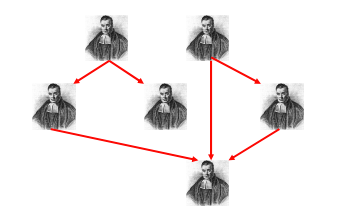Figure 1: Thomas Bayes (1701–1761) 

Bayesian Networks (BNs) are a member of probabilistic graphical models for modeling uncertainty. BN is a powerful tool for subjective logic . Bayes nets are also useful for representing flexible applicability . BN is a directed acyclic graph (DAG) where the nodes denote the random variables and the edges represent their conditional dependencies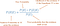Equation 1: Bayes’ Theorem 

Equation 1 illustrates Bayesian inference with Boolean variables. In this equation, p(H) denotes the prior probability of an issue belonging to class H (hypothesis) where p(E|H) is the likelihood of observing E (evidence) when the given issue belongs to H. Note that p(E|H) is mostly never equal to p(H|E). You can check on this site to remember concepts of prior, posterior, likelihood, and evidence.

Example of an intuitive explanation of Bayes’ Rule (by Michael Hochster, Ph.D. in Statistics, Stanford):

Your roommate, who’s a bit of a slacker, is trying to convince you that money can’t buy happiness, citing a Harvard study showing that only 10% of happy people are rich.

After giving it some thought, it occurs to you that this statistic isn’t very compelling. What you really want to know is what percent of rich people are happy. This would give a better idea of whether becoming rich might make you happy.

Bayes’ Theorem tells you how to calculate this other, reversed statistic using two additional pieces of information:

1. The percent of people overall who are happy

The key idea of Bayes’ theorem is reversing the statistic using the overall rates. It says that the fraction of rich people who are happy is the fraction of happy people who are rich, times the overall fraction who are happy, divided by the overall fraction who are rich.

So if

1. 40% of people are happy; and

And if the Harvard study is correct, then the fraction of rich people who are happy is:
10%×40%5%=80%10%×40%5%=80%
So a pretty strong majority of rich people are happy.

It’s not hard to see why this arithmetic works out if we just plug in some specific numbers. Let’s say the population of the whole world is 1000, just to keep it easy. Then Fact 1 tells us there are 400 happy people, and the Harvard study tells us that 40 of these people are rich. So there are 40 people who are both rich and happy. According to Fact 2, there are 50 rich people altogether, so the fraction of them who are happy is 40/50, or 80%.

Conditional dependency relations (arcs) from node A to another node B represent that node B is a child of node A or put it differently the node A is a parent of node B such in Figure 2.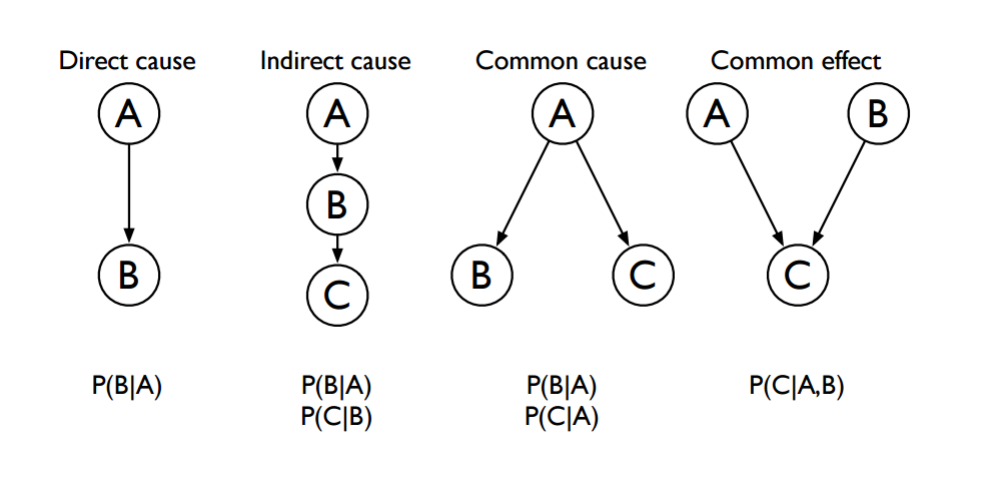Figure 2: Types of probabilistic relationships 

Each node has a prior or conditional probability distribution (cpd) according to its structure (topology). Graph structure supports the representation of knowledge, distributed algorithms for inference and learning, and intuitive interpretation.

Figure 2 demonstrates the relationship between nodes. For instance, for the common effect network configuration, we have p(A, B, C) = p(C|A, B). That is two causes of one effect which is also known as a v-structure. Let’s assume that node A represents pollution, node B is smoking and node C represents lung cancer. So, the probability of lung cancer is dependent on whether the patient smokes and the amount of pollution in the patient’s home.

The alarm network is the well-known application of a real Bayesian network. Figure 3 shows the alarm example.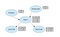Figure 3: The graphical structure of the alarm example network with probability values.

In this network, global semantics defines the full joint distribution as the product of the local conditional distributions:

We can calculate probabilities according to the given network. For instance:

• p(J, M, A,¬ B, ¬E) = p(J|A).p(M|A).p(A|¬B,¬ E).p(¬B).p(¬E)

There are some real-world applications, which are representing Bayesian Networks.

• Document Classification,

*For instance, search accuracy can be improved by understanding searcher intent and the contextual meaning of terms using Bayesian Networks. That is to search something take into consideration it’s semantic.

In addition to these applications, there are some works that are using Bayesian networks to predict the outcome of sports matches [7, 8].

## Implementation

Let’s create a simple Bayesian Networks with Jayes which is a Bayesian Network Library for Java . Jayes is implemented by Michael Kutschke as his bachelor’s thesis.

In this example, I create a Bayesian Network with three nodes. These have two, two, and three outcomes, respectively. The outcomes can be a binary such as (true, false) or (good, normal, bad). In this network, I use a common effect configuration. The classNode is conditionally dependent on the firstNode and the secondNode.

We can also compute a posterior probability using Bayes’ theorem. Here is a simple example:

## Bonus

• You can use software to create Bayesian networks and make an analysis. Weka is a popular data mining software in Java that has various Bayesian network classifier learning algorithms .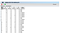Figure 4: Sample data

Figure 4 demonstrates the sampling data. We have five attributes (nodes: f1, f2, f3, f4, and f5) and their discrete values (0 or 1). The values of the class node represent the behavior of information sources, which are {honest, flip, random}.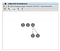Figure 5: Using Bayes Net classifier and K2 algorithm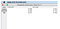Figure 6: The probability values of the first-feature node for each behavior type of information sources
• Visualizations of Bayes’ theorem,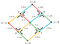Figure 7: p(A|B).p(B) = p(A, B) = p(B|A).p(A)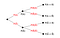Figure 8: Tree diagram for events A and B 

## Further Readings

If you are interested in this topic, I would like to recommend some resources. Bold ones are more popular than others.

## Notes

• , ” denotes AND,

If you discover any bugs in the implementation parts or if you have any questions, please do not hesitate to write them as a comment.

## Acknowledgment

Many thanks to Burak Suyunu and Yusuf Hakan Kalaycı for their reviews. This post became more understandable after their comments.

That’s all for now. I hope it will be helpful to you. Goodbye until next time!🦄

## References

 Wikipedia: Thomas Bayes

 A. Jøsang. Subjective logic. Draft book in preparation, July 2011

 L. De Raedt and K. Kersting. Probabilistic logic learning. ACM SIGKDD Explorations Newsletter, pages 31–48, 2003

 M. S. Lewicki. Artifial Intelligence Bayes Nets-I Lecture Notes. Carnegie Mellon University, 2007

 Karlis, Dimitris, and Ioannis Ntzoufras. Bayesian modelling of football outcomes: using the Skellam’s distribution for the goal difference. IMA Journal of Management Mathematics 20.2 (2008): 133–145

 Rue, Havard, and Oyvind Salvesen. Prediction and retrospective analysis of soccer matches in a league. Journal of the Royal Statistical Society: Series D (The Statistician)49.3 (2000): 399–418

 Jayes

 My M. Sc. Thesis

 Wikipedia: Bayes’ Theorem

Ph.D. Cand. in CmpE @Boğaziçi University. #ai #privacy #uncertainty #ml #dl #running #cycling #she/her https://www.cmpe.boun.edu.tr/~gonul.ayci/

## More from Gönül Aycı

Ph.D. Cand. in CmpE @Boğaziçi University. #ai #privacy #uncertainty #ml #dl #running #cycling #she/her https://www.cmpe.boun.edu.tr/~gonul.ayci/